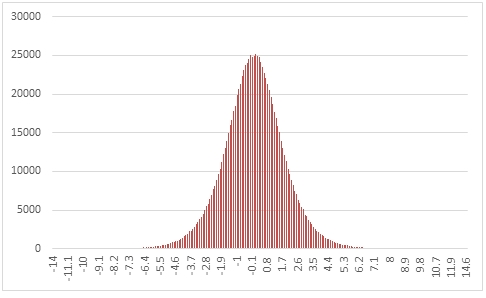# SQL Server random logistic distribution

RANDLOGISTIC

Updated: 31 March 2014

Use the table-valued function RANDLOGISTIC to generate a sequence of random numbers from a logistic distribution with parameters @Location and @Scale.
Syntax
SELECT * FROM [wctMath].[wct].[RANDLOGISTIC](
<@Rows, int,>
,<@Location, float,>
,<@Scale, float,>)
Arguments
@Rows
the number of rows to generate. @Rows must be of the type int or of a type that implicitly converts to int.
@Location
the location parameter. @Shape must be of the type float or of a type that implicitly converts to float.
@Scale
the scale parameter. @Scale must be of the type float or of a type that implicitly converts to float.
Return Types
RETURNS TABLE (
[Seq] [int] NULL,
[X] [float] NULL
)
Remarks
·         @Scale must be greater than zero.
·         If @Shape is NULL then @Shape is set to 0.
·         If @Scale is NULL then @Scale is set to 1.
·         If @Rows is less than 1 then no rows are returned.
Examples
In this example we create a sequence of 1,000,000 random numbers rounded to one decimal place from the logistic distribution with @Location = 0 and @Scale =1, COUNT the results, and paste them into Excel, and graph them.
SELECT
X,
COUNT(*) as [COUNT]
FROM (
SELECT
ROUND(X,1) as X
FROM
wct.RANDLOGISTIC(
1000000, --@Rows
0,       --@Location
1        --@Scale
)
)n
GROUP BY
X
ORDER BY
X

This produces the following result.In this example we generate 1,000,000 random numbers from a logistic distribution with @Location of 60 and @Scale of 1.333333. We calculate the mean, standard deviation, skewness, and excess kurtosis from the resultant table and compare those values to the expected values for the distribution.
DECLARE @size as int = 1000000
DECLARE @location as float = 60
DECLARE @scale as float = 1.333333
DECLARE @mean as float = @location
DECLARE @var as float = POWER(@scale,2)*POWER(PI(),2)/3e+00
DECLARE @stdev as float = SQRT(@var)
DECLARE @skew as float = 0
DECLARE @kurt as float = 1.2

SELECT
stat,
[RANDLOGISTIC],
[EXPECTED]
FROM (
SELECT
x.*
FROM (
SELECT
AVG(x) as mean_LOGISTIC,
STDEVP(x) as stdev_LOGISTIC,
wct.SKEWNESS_P(x) as skew_LOGISTIC,
wct.KURTOSIS_P(x) as kurt_LOGISTIC
FROM
wct.RANDLOGISTIC(@size,@location,@scale)
)n
CROSS APPLY(
VALUES
('RANDLOGISTIC','avg', mean_LOGISTIC),
('RANDLOGISTIC','stdev', stdev_LOGISTIC),
('RANDLOGISTIC','skew', skew_LOGISTIC),
('RANDLOGISTIC','kurt', kurt_LOGISTIC),
('EXPECTED','avg',@mean),
('EXPECTED','stdev',@stdev),
('EXPECTED','skew',@skew),
('EXPECTED','kurt',@kurt)
)x(fn_name,stat,val_stat)
)d
PIVOT(sum(val_stat) FOR fn_name in([RANDLOGISTIC],[EXPECTED])) P

This produces the following result (your result will be different).

 stat RANDLOGISTIC EXPECTED avg 59.9978527 60 kurt 1.211283085 1.2 skew 0.001022549 0 stdev 2.413409367 2.418398548

### SupportCopyright 2008-2023 Westclintech LLC         Privacy Policy        Terms of Service